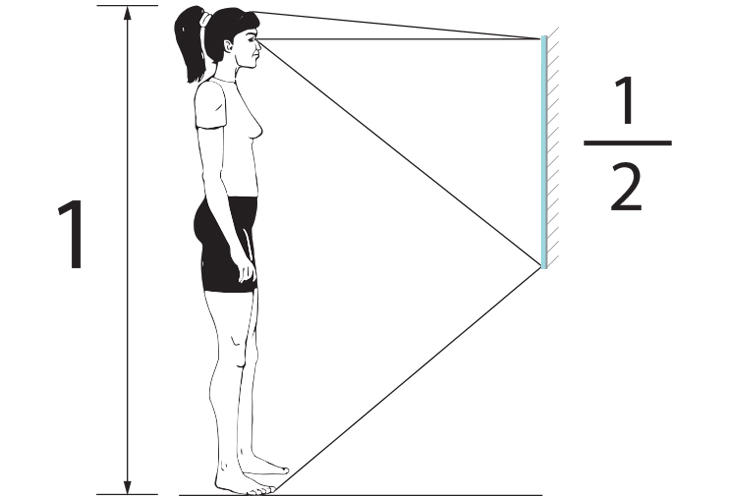# Shortest mirror to see full length of your body

In the diagram below, what is the shortest mirror that would allow you to see the full length of your body however far away you stand from the mirror?

The answer is half the height of yourself, and we will prove that as follows!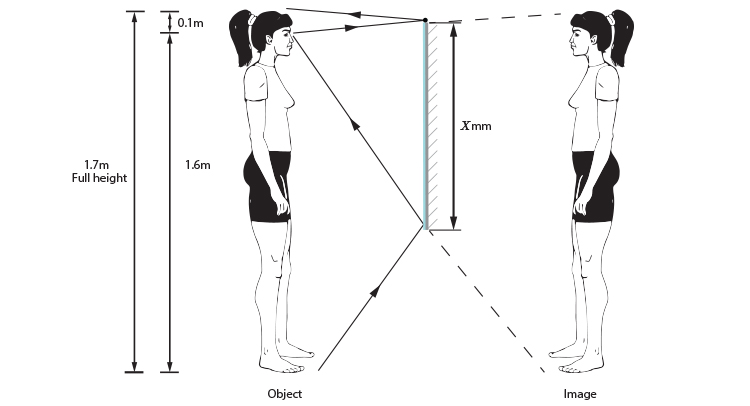The best way to tackle this problem is to redraw the diagram in a simpler format. Use a dot for the bottom and top of the drawn head and use a single eye as the observation point. Initially put in a mirror the same height as the observer, one metre away from the observer.

We would get the following ray diagram: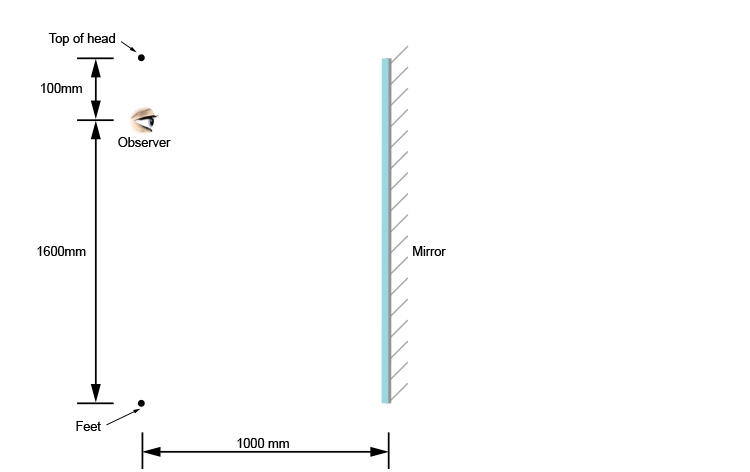Now apply the laws of reflection.

The first stage of this is to draw an image on the other side of the mirror parallel to the mirror.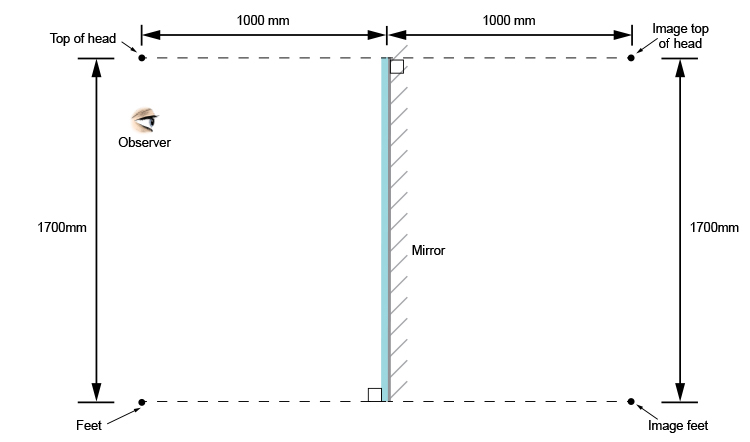You should end up with a diagram as follows: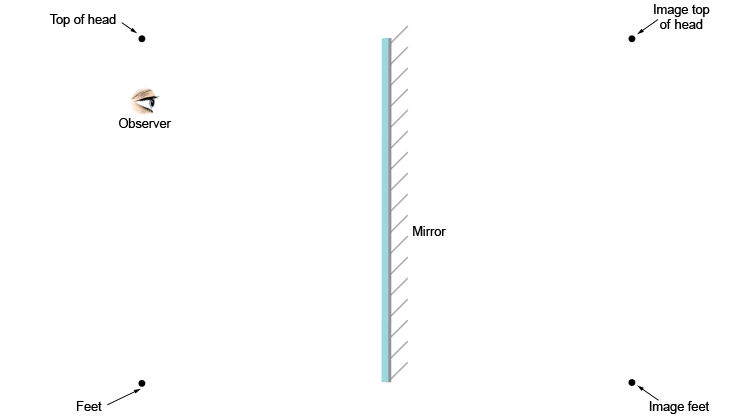The second stage is to draw rays to the observer point from each of the image points. We can also complete the third stage by joining the light rays from the mirror to the object points as follows: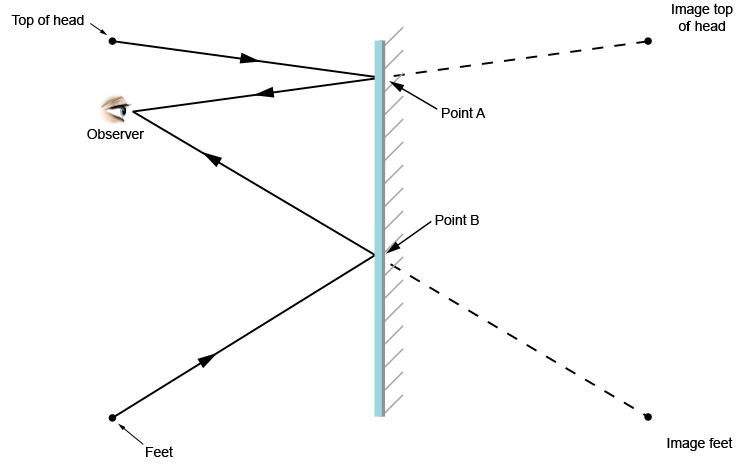You can see that the observer can see an image of the top of their head and an image of their feet.

Anything above point A or below point B is unnecessary.

But where is point A? Let's look at a blow-up of point A.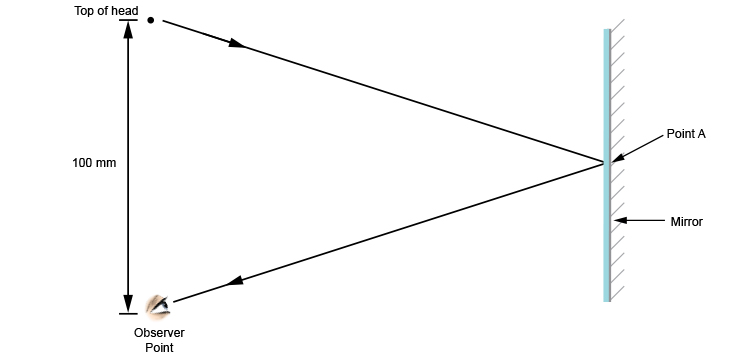If we remember the three laws of reflection, then key to this would be that:

The angle of incidence = the angle of reflection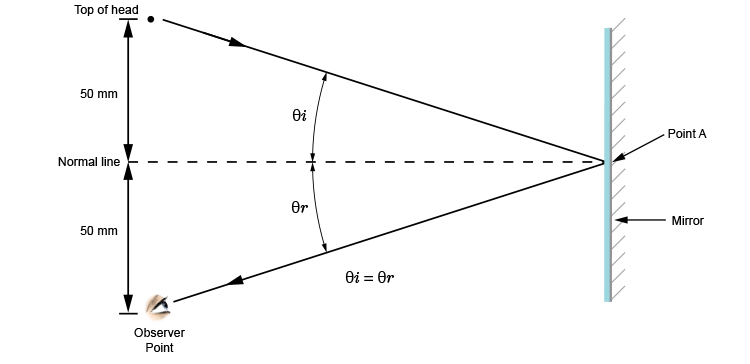In order that the angle of incidence equals the angle of reflection, the normal line must sit exactly half-way between the top of the head and the observer point.

We therefore don't need the top edge of the mirror to be higher than 50mm above the observer point.

So now let's look at a blow-up of point B.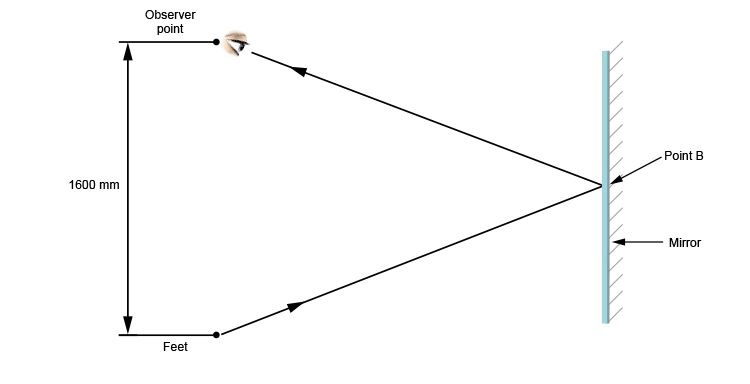Again, remembering the most important aspect of the three laws of reflection:

The angle of incidence = the angle of reflection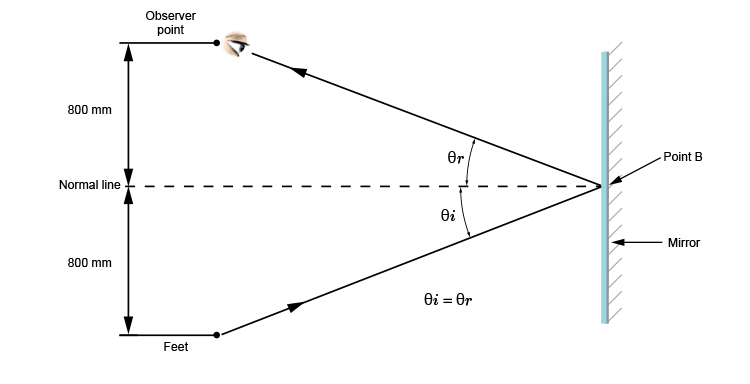In order that the angle of incidence equals the angle of reflection, the normal line must sit exactly half-way between the observation point and the feet.

We therefore don't need the bottom edge of the mirror to be lower than 800mm from the floor.

From this we would get: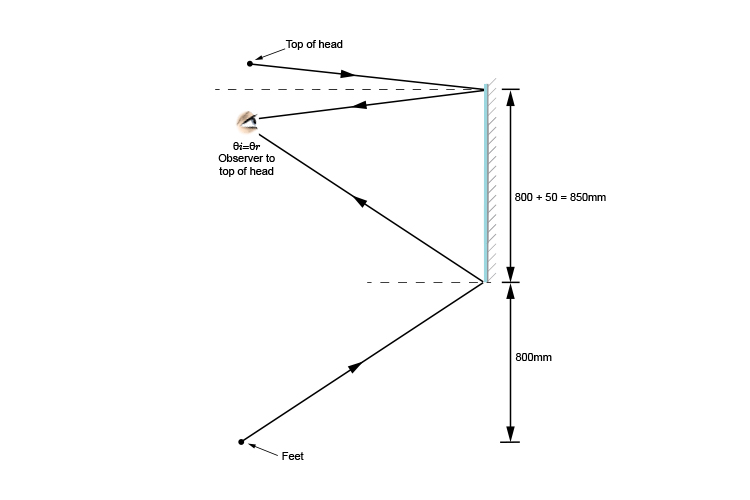The observer is 1700mm high and the mirror needs to be 850mm long and, yes, this is half the height.

Not only that, but we can state that the bottom of the mirror needs to start at 800mm from the floor.

But does this work for any distance that the observer stands away from the mirror?

What if the person were standing within 200mm?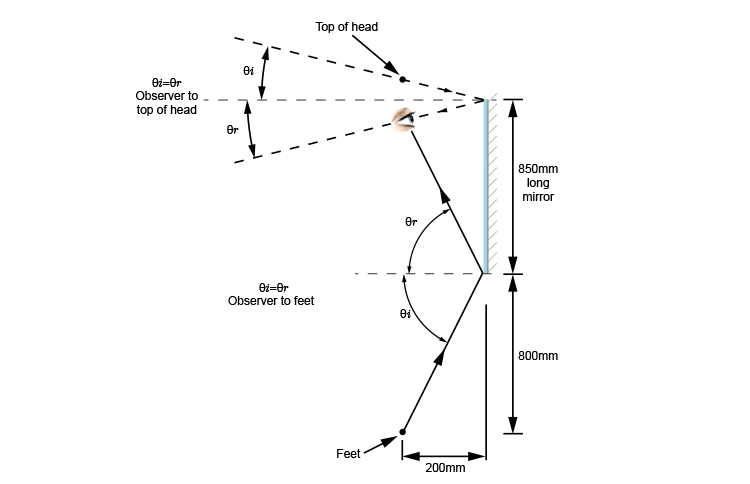We can see that this still works for a very close distance to the mirror. What about at a very long distance apart?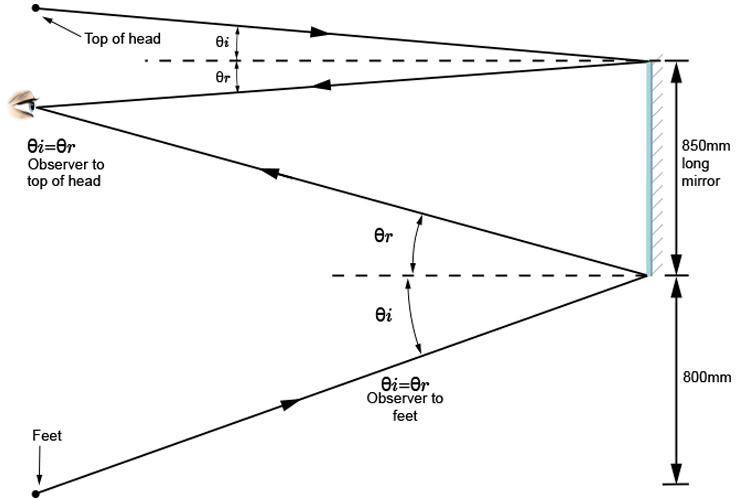The mirror length of 850mm still works when the observer is very far away from the mirror.

## Summary

It doesn't matter how far the observer is from the mirror

The mirror must be 850mm long

The mirror needs to start at 800mm from the floor

NOTE: Obviously if you are only 50mm from the mirror the only way to see your feet is to tilt your head and then your feet are not in alignment and the above diagram does not then apply.

## Conclusion

The mirror to purchase for any observer to see their full height needs to be a minimum of## Remember